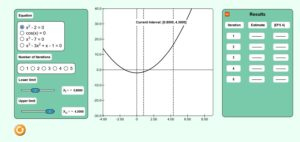# Chapter 3 Bisection Method Simulation

Description:  This simulation depicts the bisection method of solving nonlinear equations.  The student can explore the way the root estimate converges, and also note the rate of convergence.  They can also simulate the pitfalls of the bisection method.Keywords:  roots of nonlinear equations, bisection method.

Learning Objectives:  After successful completion of running the simulation with proper questions asked by the instructor, the student would be able to 1) note the convergence of the method 2) the bracketing nature of the method, 3) rate of convergence, 4) study the pitfalls of the method.

Full Resources:  The full resources for the topic of the bisection method are given here which include textbook content, a PowerPoint presentation, a multiple-choice test, audiovisual lectures, and application examples.